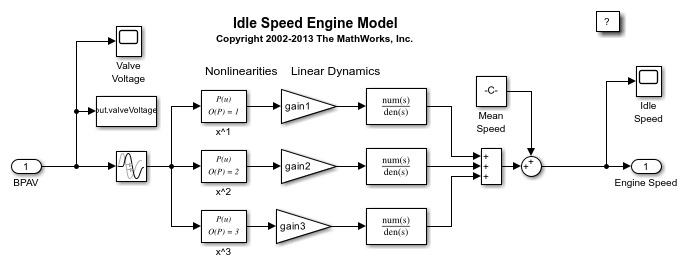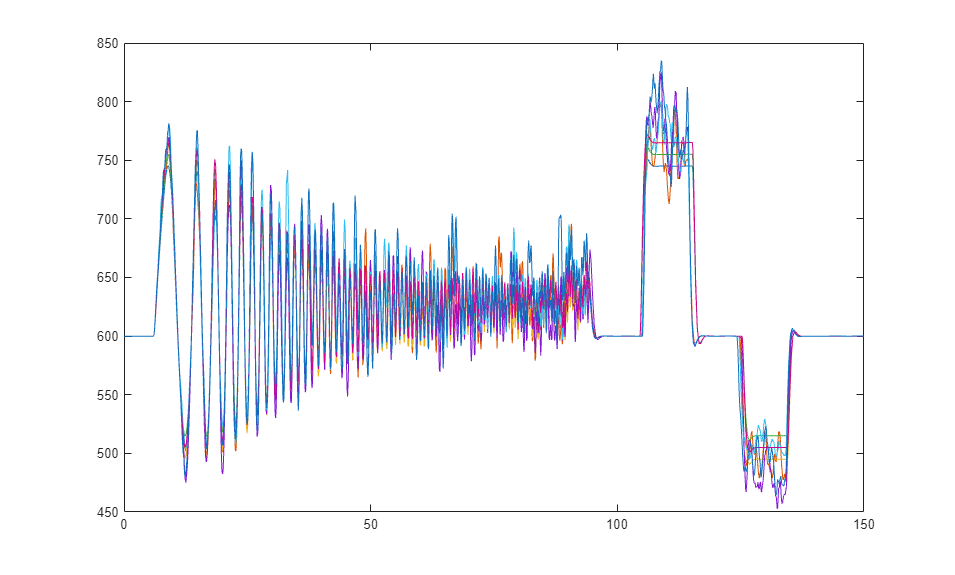# Rapid Accelerator Simulations Using Parsim

This example shows the use of Rapid Accelerator in applications that require running parallel simulations for a range of input and parameter values.

The example uses a model that simulates the idle speed of an engine. The model input is the voltage of the bypass air valve. The output is the idle speed.

Use `parsim` to run parallel simulations with two sets of valve voltages. Independently vary two of the three transfer function gain parameters over a range of two values. The following table lists the eight simulations, along with the parameter values. In Step 2, create the external inputs, `inpSets`. The variables `gain2` and `gain3` correspond to the two gain parameters.

` Run 1 inpSets(1) gain2 = 25 gain3 = 20`

` Run 2 inpSets(1) gain2 = 25 gain3 = 30`

` Run 3 inpSets(1) gain2 = 35 gain3 = 20`

` Run 4 inpSets(1) gain2 = 35 gain3 = 30`

` Run 5 inpSets(2) gain2 = 25 gain3 = 20`

` Run 6 inpSets(2) gain2 = 25 gain3 = 30`

` Run 7 inpSets(2) gain2 = 35 gain3 = 20`

` Run 8 inpSets(2) gain2 = 35 gain3 = 30`

### Step 1: Preparation

First, open the model. The simulation mode is set to Rapid Accelerator. The default input data, and the required parameters are preloaded in the base workspace.

Open model:

```mdl = 'sldemo_raccel_engine_idle_speed'; open_system(mdl); ```### Step 2: Create Input Sets

Perturb the default input values vector to obtain a new input values vector.

```inpSets(1) = timeseries(inpData, time); rndPertb = 0.5 + rand(length(time), 1); inpSets(2) = timeseries(inpData.*rndPertb, time); numInpSets = length(inpSets); ```

### Step 3: Create Parameter Sets

Next, examine how the idle speed changes for different values of parameters `gain2` and `gain3`. Create an array of `Simulink.SimulationInput` objects to specify the different parameter values and external input for each simulation. The array of SimulationInput objects is preallocated for better performance. Note that you can directly specify the external input on the `SimulationInput` object instead of using a model parameter.

```gain2_vals = 25:10:35; gain3_vals = 20:10:30; num_gain2_vals = length(gain2_vals); num_gain3_vals = length(gain3_vals); numSims = num_gain2_vals*num_gain3_vals*numInpSets; in(1:numSims) = Simulink.SimulationInput(mdl); idx = 1; for iG2 = 1:num_gain2_vals for iG3 = 1:num_gain3_vals for inpSetsIdx = 1:numInpSets in(idx) = in(idx).setModelParameter('SimulationMode', 'rapid', ... 'RapidAcceleratorUpToDateCheck', 'off', ... 'SaveTime', 'on', ... 'SaveOutput', 'on'); % Use setVariable to specify a new value for a variable during % simulations in(idx) = in(idx).setVariable('gain2', gain2_vals(iG2)); in(idx) = in(idx).setVariable('gain3', gain3_vals(iG3)); in(idx).ExternalInput = inpSets(inpSetsIdx); idx = idx + 1; end end end ```

Note: This example uses the `setModelParameter` method of the `SimulationInput` object to set model parameters to run the simulations in Rapid Accelerator mode and enable logging. The Rapid Accelerator target is built using the `SetupFcn`. The Rapid Accelerator target is built once and used by all subsequent simulations, saving the time required for model compilation. Here is the code for the SetupFcn

```function sldemo_parallel_rapid_accel_sims_script_setup(mdl) % Temporarily change the current folder on the workers to an empty % folder so that any existing slprj folder on the client does not % interfere in the build process. currentFolder = pwd; tempDir = tempname; mkdir(tempDir); cd (tempDir); oc = onCleanup(@() cd (currentFolder)); Simulink.BlockDiagram.buildRapidAcceleratorTarget(mdl); end ```

### Step 4: Execute Simulations

Use the `parsim` function to execute the simulations in parallel. The array of `SimulationInput` objects, `in`, created in the last step is passed into the `parsim` function as the first argument. Store the simulation output data in a variable, `out`, whose value is an array of `Simulink.SimulationOutput` objects. Each `SimulationOutput` object contains the logged signal along with the `SimulationMetadata`. When running multiple simulations using `parsim`, errors are captured so that subsequent simulations can continue to run. Any errors would show up in the `ErrorMessage` property of the `SimulationOutput` object.

```out = parsim(in, 'ShowProgress', 'on', ... 'SetupFcn', @() sldemo_parallel_rapid_accel_sims_script_setup(mdl)); ```
```[21-May-2021 17:46:47] Checking for availability of parallel pool... Starting parallel pool (parpool) using the 'local' profile ... Connected to the parallel pool (number of workers: 6). [21-May-2021 17:47:51] Starting Simulink on parallel workers... Analyzing and transferring files to the workers ...done. [21-May-2021 17:48:54] Configuring simulation cache folder on parallel workers... [21-May-2021 17:48:55] Running SetupFcn on parallel workers... [21-May-2021 17:50:14] Loading model on parallel workers... [21-May-2021 17:50:22] Running simulations... [21-May-2021 17:50:29] Completed 1 of 8 simulation runs [21-May-2021 17:50:29] Completed 2 of 8 simulation runs [21-May-2021 17:50:29] Completed 3 of 8 simulation runs [21-May-2021 17:50:29] Completed 4 of 8 simulation runs [21-May-2021 17:50:29] Completed 5 of 8 simulation runs [21-May-2021 17:50:29] Completed 6 of 8 simulation runs [21-May-2021 17:50:36] Completed 7 of 8 simulation runs [21-May-2021 17:50:36] Completed 8 of 8 simulation runs [21-May-2021 17:50:36] Cleaning up parallel workers... ```

### Step 5: Plot Results

Plot the engine idle speed with respect to time for different parameter values and inputs. The output is logged in the array format and can be accessed from the SimulationOutput object.

```for i = 1:numSims simOut = out(i); t = simOut.tout; y = simOut.yout; plot(t, y) hold all end ```### Step 6: Close MATLAB Workers

```delete(gcp('nocreate')) ```